Courses

# Previous year Questions (2016-20) - Structure of Atom Notes | EduRev

## JEE : Previous year Questions (2016-20) - Structure of Atom Notes | EduRev

The document Previous year Questions (2016-20) - Structure of Atom Notes | EduRev is a part of the JEE Course Chemistry for JEE.
All you need of JEE at this link: JEE

Q.1. The number of orbitals associated with quantum number n = 5, m= +1/2 is    (2020)
(1) 11
(2) 25
(3) 50
(4) 15
Ans.
(2)
For principle quantum number (n) = 5, total of 5 subshells are associated that is s, p, d, f and g-subshells. Each orbital has two spin quantum number (ms) = + 1/2 and 1/2. Thus, number of orbitals associated with n = 5 and m= + 1/2 are equal to the number of orbitals in 5th shell.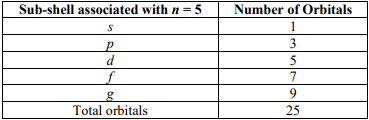Q.2. For the Balmer series in the spectrum of H atom,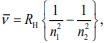the correct statements among (I) to (IV) are    (2020)
(I) As wavelength decreases, the lines in the series converge
(II) The integer n1 is equal to 2
(III) The lines of longest wavelength correspond to n2 = 3
(IV) The ionization energy of hydrogen can be calculated from wave number of these lines
(1) (I), (III), (IV)
(2) (I), (II), (III)
(3) (I), (II), (IV)
(4) (II), (III), (IV)
Ans.
(2)
For Balmer series in hydrogen spectrum, as the wavelength decreases, the lines in the series get converge. For Balmer series, n1 is 2 and for longest wavelength n2 is 3.

Q.3. The radius of the second Bohr orbit, in terms of the Bohr radius, a0, in Li2+ is    (2020)
(1) 2a0/9
(2) 4a0/9
(3) 4a0/3
(4) 2a0/9
Ans.
(3)
We know,
Radius of Bohr’s orbit = a0n2/Z
For Li2+ (Z = 3), the radius of second orbit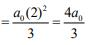Q.4. The de Broglie wavelength of an electron in the 4th Bohr orbit is    (2020)
(1) 2πa0
(2) 4πa0
(3) 6πa0
(4) 8πa0
Ans.
(4)
From the relation,
2πr = nλ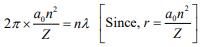For hydrogen atom,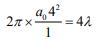⇒ λ = 8πa0

Q.5. For emission line of atomic hydrogen from ni = 8 to n= n, the plot of wave number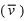against (1/n2) will be (The Rydberg constant, RH is in wave number unit)    (2019)
(1) Linear with intercept - RH
(2) Non linear
(3) Linear with slope RH
(4) Linear with slope - RH
Ans.
(4)
As we know,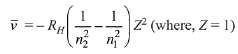After putting the values, we get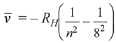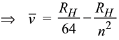Comparing to y = mx + c, we get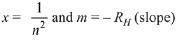Q.6. Which of the following combination of statements is true regarding the interpretation of the atomic orbitals?
(a) An electron in an orbital of high angular momentum stays away from the nucleus than an electron in the orbital of lower angular momentum.
(b) For a given value of the principal quantum number, the size of the orbit is inversely proportional to the azimuthal quantum number.
(c) According to wave mechanics, the ground state angular momentum is equal to  h/2π
(d) The plot of ψ vs r for various azimuthal quantum numbers, shows peak shifting towards higher r value.    (2019)
(1) (a), (d)
(2) (a), (b)
(3) (a),(c)
(4) (b), (c)
Ans.
(1)
(a) Angular momentum (L) = nh/2π
Therefore, as n increases, L also increases.
(b)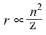(c) For n = 1, L = 4/2π
(d) As l increases, the peak of ψ vs r shifts towards higher 'r' value.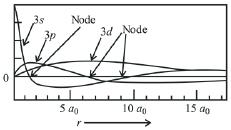Q.7. Which of the graphs shown below does not represent the relationship between incident light and the electron ejected from metal surface?    (2019)
(1)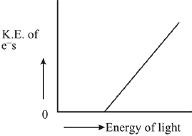(2)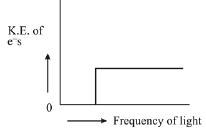(3)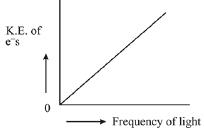(4)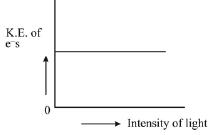Ans.
(3)
K.E. = hv - hv0
where, v = Frequency of incident radiation
v0 = Threshold frequency
KE is independent of intensity, it depends on frequency of light. Intensity is directly proportional to the no. of electrons emitted.

Q.8. The ground state energy of hydrogen atom is -13.6 eV. The energy of second excited state of He+ ion in eV is:    (2019)
(1) -54.4
(2) -3.4
(3) -6.04
(4) -27.2
Ans.
(3)
According to Bohr’s model energy in nth state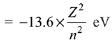For second excited state, of He+, n = 3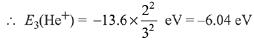Q.9. Heat treatment of muscular pain involves radiation of wavelength of about 900 nm. Which spectral line of H-atom is suitable for this purpose?
[RH = 1 x 105 cm-1. h = 6.6 x 10-34 Js, c = 3 x 108 ms-1]    (2019)
(1) Paschen,∞ → 3
(2) Paschen, 5 → 3
(3) Balmer, ∞ → 2
(4) Lyman, ∞ → 1
Ans.
(1)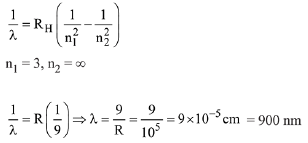Q.10. The de Broglie wavelength (λ) associated with a photo-electron varies with the frequency (v) of the incident radiation as, [v0 is threshold frequency]:    (2019)
(1)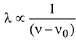(2)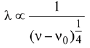(3)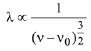(4)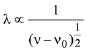Ans.
(4)
According to de-Broglie wavelength equation,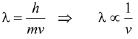According to photoelectric effect,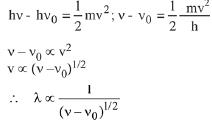Q.11. What is the work function of the metal if the light of wavelength 4000 Å generates photo-electrons of velocity 6 x 105 ms-1 from it?    (2019)
(Mass of electron= 9 x 10-31 kg
Velocity of light = 3 x 108 ms-1
Planck’s constant= 6.626 x 10-34 Js
Charge of electron = 1.6 x 10-19 JeV-1)
(1) 0.9 eV
(2) 3.1 eV
(3) 2. leV
(4) 4.0 eV
Ans.
(3)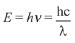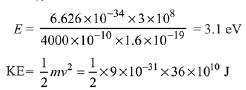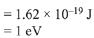According to photoelectric effect,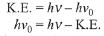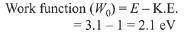Q.12. If the de Broglie wavelength of the electron in nth Bohr orbit in a hydrogenic atom is equal to 1.5 πa0 (a0 is Bohr radius), then the value of n/z is:    (2019)
(1) 0.40
(2) 1.50
(3) 1.0
(4) 0.75
Ans.
(4)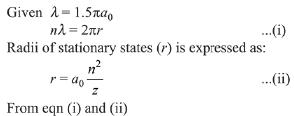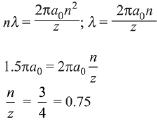Q.13. The quantum number of four electrons are given below:
I. n = 4, l = 2, m1 = -2, ms = -1/2
II. n = 3, l = 2, m1= 1, m= + 1/2
III. n = 4, l = 1, m1 = 0,  ms = + 1/2
IV. n = 3, l= 1, m1= 1, ms = - 1/2
The correct order of their increasing energies will be:    (2019)
(1) IV<III<II<I
(2) I<II<III<IV
(3) IV<II<III<I
(4) I<III<II<IV
Ans.
(3)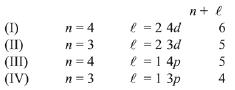The energy of an atomic orbital increases with increasing n + l. For identical values of n + l, energy increases with increasing n. Therefore the correct order of energy is: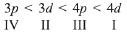Q.14. If p is the momentum of the fastest electron ejected from a metal surface after the irradiation of light having wavelength λ, then for 1.5p momentum of the photoelectron, the wavelength of the light should be:
(Assume kinetic energy of ejected photoelectron to be very high in comparison to work function):    (2019)
Ans.
(4)
In photoelectric effect,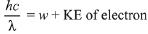Given that KE of ejected photoelectron is very high in comparison to work function w.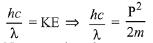New wavelength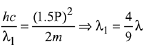Q.15. For any given series of spectral lines of atomic hydrogen, let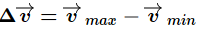be the difference in maximum and minimum frequencies in cm-1. The ratio of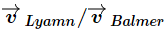is:   (2019)
(1) 4 : 1
(2) 9 : 4
(3) 5 : 4
(4) 27 : 5
Ans.
(2)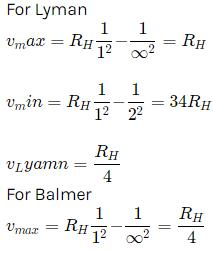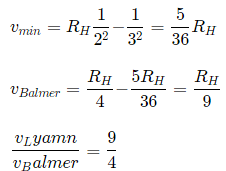Q.16. Which one of the following about an electron occupying the 1s orbital in a hydrogen atom is incorrect? (The Bohr radius is represented by a0).
(1) The probability density of finding the electron is maximum at the nucleus.
(2) The electron can be found at a distance 2a0 from the nucleus.
(3) The magnitude of the potential energy is double that of its kinetic energy on an average.
(4) The total energy of the electron is maximum when it is at a distance a0 from the nucleus.    (2019)
Ans.
(4)
The total energy of the electron is minimum at a distance of a0 from the nucleus for 1s orbital.

Q.17. The graph between |ψ|2 and r (radial distance) is shown below. This represents:    (2019)
(1) 3s orbital
(2) 2s orbital
(3) 1s orbital
(4) 2p orbital
Ans.
(2)
The given probability density curve is for 2s orbital due to the presence of only one radial node. 1s and 2p orbital do not have any radial node and 3s orbital has two radial nodes. Hence, option (2) is correct.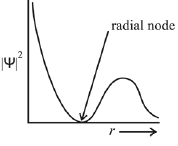Q.18. The isoelectronic set of ions is:    (2019)
(1) N3-, O2-, F- and Na+
(2) N3 Li+, Mg2+ and O2-
(3) F-, Li+, Na+ and Mg2+
(4) Li+, Na+, O2- and F-
Ans.
(1)
Atomic numbers of N, O, F and Na are 7, 8, 9 and 11 respectively. Therefore, total number of electrons in each of N3-, O2-, F-, and Na+ are 10 and hence they are isoelectronic.

Q.19. The ratio of the shortest wavelength of two spectral series of hydrogen spectrum is found to be about 9. The spectral series are:    (2019)
(1) Lyman and Paschen
(2) Balmer and Brackett
(3) Brackett and P fund
(4) Paschen and P fund
Ans.
(1)
For determined shortest wavelength, n2 = ∞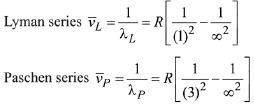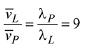Q.20. The group number, number of valence electrons, and valency of an element with atomic number 15, respectively, are:    (2019)
(1) 16, 5 and 2
(2) 15, 5 and 3
(3) 16, 6 and 3
(4) 15, 6 and 2
Ans.
(2)
Phosphorus has atomic number 15. Its group number is 15, number of valence electrons is 5 and valency is 3.

Q.21. The electrons are more likely to be found:    (2019)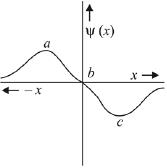(1) in the region a and c
(2) in the region a and b
(3) only in the region a
(4) only in the region c
Ans.
(1)
Probability of finding an electron will have maximum value at both 'a' and 'c'. There is zero probability of finding an electron at 'b'.

Q.22. Ejection of the photoelectron from metal in the photoelectric effect experiment can be stopped by applying 0.5 V when the radiation of 250 nm is used. The work function of the metal is:    (2018)
(1) 4.5 eV
(2) 4 eV
(3) 5.5 eV
(4) 5 eV
Ans.
(1)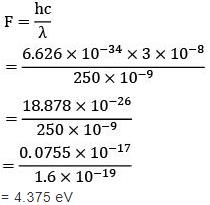Q.23. Which of the following statements is false?    (2018)
(1) Splitting of spectral lines in electrical field is called Stark effect
(2) Frequency of emitted radiation from a black body goes from a lower wavelength of higher wavelength as the temperature increases
(3) Photon has momentum as well as wavelength
(4) Rydberg constant has unit of energy
Ans.
(2, 4)
When temperature is increased, black body emit high energy radiation, from higher wavelength to lower wavelength.
Rydberg constant has unit length–1 (i.e. cm–1)

Q.24. The radius of the second Bohr orbit for hydrogen atom is
(Planck's Const. h = 6.6262 × 10–34 Js;
mass of electron = 9.1091 × 10–31 kg;
charge of electron e = 1.60210 × 10–19 C;
permittivity of vacuum ε0 = 8.854185 × 10–12 kg–1 m–3 A2)    (2017)
(1) 1.65 Å
(2) 4.76 Å
(3) 0.529 Å
(4) 2.12 Å
Ans.
(4)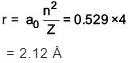Q.25. If the shortest wavelength in Lyman series of hydrogen atom is A, then the longest wavelength in Paschen series of He+ is:    (2017)
(1) 5A/9
(2) 36A/7
(3) 36A/5
(4) 9A/5
Ans.
(2)
Shortest wavelength is corresponding to best ine
∴ nL = 1 (Lyman series)
nH = ∞ (infinite)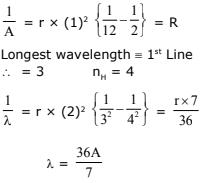Q.26. The electron in the hydrogen atom undergoes transition from higher orbitals to orbital of radius 211.6 pm. This transition is associated with:    (2017)
(1) Lyman series
(2) Balmer series
(3) Brackett series
(4) Paschen series
Ans.
(2)
R = 211.6 pm = 2.11 Å
R = 0.529 x n2/Z = 2.11 Å
n2 = 4 ⇒ n = 2

Q.27. A stream of electrons from a heated filament was passed between two charged plates kept at a potential difference V esu. If e and m are charge and mass of an electron, respectively, then the value of h/l (where l is wavelength associated with electron wave) is given by:    (2016)
(1) me V
(2) 2me V
(3)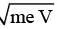(4)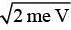Ans.
(4)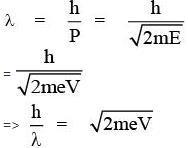Q.28. The total number of orbitals associated with the principal quantum number 5 is:    (2016)
(1) 5
(2) 20
(3) 25
(4) 10
Ans.
(3)
Given, n = 5
Possible subshells are
5s, 5p, 5d, 5f, 5g
Total number of orbital = 1 + 3 + 5 + 7 + 9 = 25

Q.29. Aqueous solution of which salt will not contain ions with the electronic configuration 1s2 2s2 2p6 3s2 3p6?    (2016)
(1) NaCl
(2) CaI2
(3) NaF
(4) KBr
Ans.
(3)
NaF: Na+ = 1s2s2p6
F = 1s2s2p6

Offer running on EduRev: Apply code STAYHOME200 to get INR 200 off on our premium plan EduRev Infinity!

## Chemistry for JEE

223 videos|452 docs|334 tests

,

,

,

,

,

,

,

,

,

,

,

,

,

,

,

,

,

,

,

,

,

;